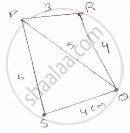Share
Notifications

View all notifications
Books Shortlist
Your shortlist is empty

# Find the Area of a Quadrilateral Abcd is Which Ab = 3 Cm, Bc = 4 Cm, Cd = 4 Cm, Da = 5 Cm and Ac = 5 Cm. - CBSE Class 9 - Mathematics

Login
Create free account

Forgot password?
ConceptApplication of Heron’S Formula in Finding Areas of Quadrilaterals

#### Question

Find the area of a quadrilateral ABCD is which AB = 3 cm, BC = 4 cm, CD = 4 cm, DA = 5 cm and AC = 5 cm.

#### Solution

For ΔPQRPQ^2=QR^2+RP^2

(5^2)=(3)^2+(4)^2        [∵𝑃𝑅=3 𝑄𝑅=4 𝑎𝑛𝑑 𝑃𝑄=5]
So, ΔPQR is a right angled triangle. Right angle at point R.

Area of ΔABC =1/2xxQRxxRP

=1/2xx3xx4

=6cm^2

For ΔQPS
Perimeter = 2s = AC + CD + DA = (5 + 4 + 5)cm = 14 cm
S = 7 cm
By Heron’s formulae

Area of Δlesqrt(s(s-a)(s-b)(s-c))cm^2

Area of Δle PQS =sqrt(7(7-5)(7-4)(7-3))cm^2

=2sqrt(21)cm^2

=(2xx4.583)cm^2

=9.166cm^2

Area of PQRS = Area of PQR + Area of ΔPQS = (6+9.166)cm^2=15.166  cm^2

Is there an error in this question or solution?

#### APPEARS IN

Solution Find the Area of a Quadrilateral Abcd is Which Ab = 3 Cm, Bc = 4 Cm, Cd = 4 Cm, Da = 5 Cm and Ac = 5 Cm. Concept: Application of Heron’S Formula in Finding Areas of Quadrilaterals.
S